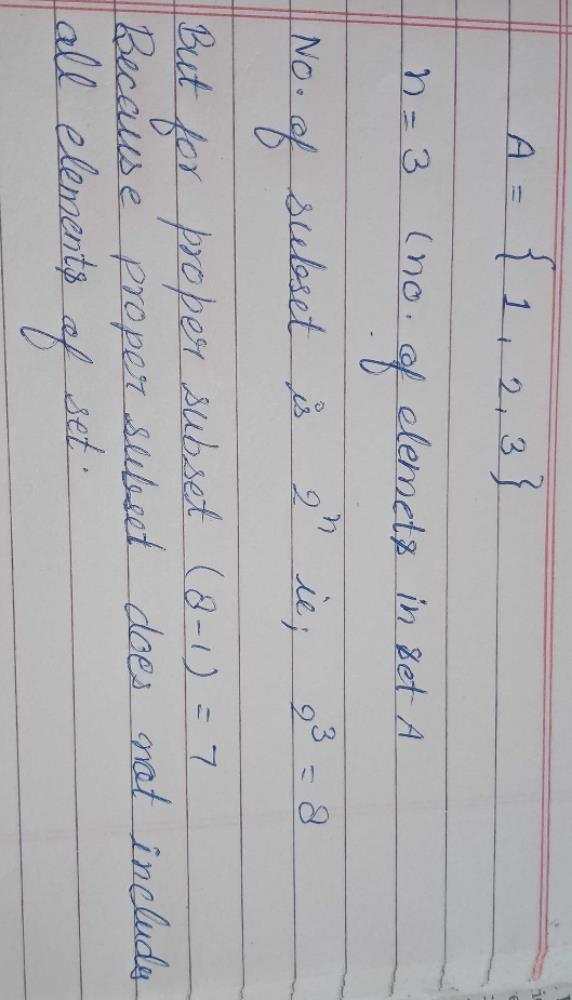Courses

# The number of proper subsets of the set { 1, 2 , 3 } is :a)7b)5c)6d)8Correct answer is 'A'. Can you explain this answer? Related Test: Test: Set Notations

## JEE Question

By Chhayank Thakur · Apr 28, 2020 ·JEE
Mysterio Man answered 3 weeks ago
Proper subset for the set {1,2,3}={},{1},{2},{3},
{1,2},{2,3},{3,1}=7 subsets

Shalini Nawariya answered Apr 28, 2020
It should be 8 ....
{1,2,3,(1,2),(1,3),(2,3),(1,2,3),fi }

Shiva Keshari answered Jul 07, 2020K.p.s Singh answered Jul 03, 2020
It should be 8,because no of subset =2^n,=2^3=8

How you put photo

Anjali Sharma answered 3 weeks ago
Ans is 8
Proper subset of A is {},{1}, {2} , {3}, {1,2},{2,3}, {1,3},{1,2,3}

Srihasa Bathula answered 2 weeks ago
{}{1}{2}{3}{1,2}{2,3}{3,1}

Khaja Moinuddin answered Jul 03, 2020
A proper subset is all subsets possible for a given set excluding the set itself so the traditional formula reduces to 2^(n)-1...so the answer for this question is 2³-1=7

Shagun Mehta answered Apr 28, 2020
Always remember the formula for finding proper set is 2^n -1 where n is no. of elements

Manish Kumar answered Jul 04, 2020
Which of the following has only one suset

Mohit Mittal answered 3 weeks ago
No. of proper subsets = 2^n - 1
are = {}, {1}, {2}, {3}, {1,2}, {2,3}, {1,3}.

Amit Gulbhile answered Jul 05, 2020
Proper subsets of any set A={m} are 2^m-1.
so proper subsets of set having 3 elements are 2^3-1=7
so the answer is option A

Sid Gupta answered May 27, 2018
6

Ritu Singh answered Feb 01, 2020
Number of subsets of a given set = 2n

Anees Mohammed answered 2 weeks ago
Total subsets = 2^n
as n=3 :total sets =8 but proper set contains atleast single element then
total subsets -1 : 8-1= 7

Ishaan Dhawan answered 5 days ago
8 subsets

Supriya Singh answered May 27, 2020
{1,2,3},{1,2},{2,3},{3,1},{1},{2},{3},{}
always remember an empty set is a subset of every set.

Omkar Rane answered Jul 08, 2020
A={1,2,3}
Therefore, n=3
Hence,
Number of subsets=2^3.
But number of proper subsets are always 1 lesser than the number of subsets.
Because proper subset does not have all the elements which are in the given set.
Hence, number of proper subsets=2^3-1
=8-1
=7
That's solve.

Lakkireddy Ramireddy answered 4 weeks ago
Proper subsets 2 power n that is 2.2.2 =8

Amrit Raj Garg answered 4 weeks ago
The basic formula for proper subset is 2^n , here n= no of elements in the set

So your answer is very simple as no of proper subsets ,2³= 8 as here n=3 ( no of elements ).

~A.r.Garg

Rekha Sharma answered 4 weeks ago
Formula for no. of subset =2^n
but for proper subset=2^n-1
so here no of elements are 3 then answer must be 7 as 2^3-1=8-1=7

Ashvin Shendge answered 2 weeks ago
Ans.(a) 7
1.{}
2.{1}
3.{2}
4.{3}
5.{1,2}
6.{2,3}
7.{1,3}

Love Kumar answered 2 hours ago
1

Martin Kumar answered 3 weeks ago
Number of proper subsets={}, {1}, {2}, {3}, {1, 2}, {1, 3}, {2, 3}

Ac Gupta answered Jun 23, 2020
Because no of subset is 2m, which is here 8 but in includes set it self which is not proper subset. So 7 is subset.